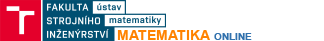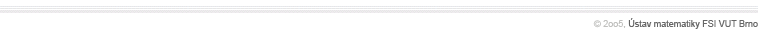Number seriesDefinition of a series, partial sums, convergent, divergent and oscillating series, necessary condition for teh convergene of a series, geometric series, sums and differences of series, series with positive termsNumber series - advanced issuesAlternating series, Leignitz convergence test for alternating series, absolutely convergent series, relatively convergent series, rearranging the terms of a relatively convergent series, Riemann theorem Convergence testsComparison test, root test, quotient test, integral testFunction sequences and seriesDefinition of a function series, point-wise convergent series, unifomly convergent series, comparing poit-wise and uniform convergence, Cauchy's test of uniform convergence, unifrm convergence of continuous functions, integrating and differentiating uniformly convergent function series, uniform convergence tets by Weierstrass and DirichletPower seriesDefinition of a power series, radius of convergence, derivative of a power series, integral of a power series, calculating Pi using Machin's identity     Taylor seriesDefinition of a power series, MacLaurin series, conditions for the convergence of a formal power series, example of a non-convergent formal Taylor series, MacLaurin series of sin(x), cos(x), exp(x), ln(1+x)